## //----------------------------// ArtMatic 2 in 3 out components //----------------------------//

Introduction
3D z set
3D Axonometric
Inverse projections #
3D plane
3D sphere
3D ellipsoid
3D tube
3D cube
3D box
3D room
3D parametric face
3D parametric cube
3D parametric room
Perlin noise + derivative
Looper
RGB linear hues
RGB concentric hues
RGB Pict/Movie
RGB Pict/Movie mirror
Pict/Movie Overlay
RGB Multi Perlin random
RGB Random Fractal
RGB MultiFractal
Lunar rocks
Color Bubbles
String noise
Techno boxes
Color Pyramids
RGB grass
RGB foliage
RGB soft random hues
Packed XYA logic #
Packed Vector Maths #
Packed RGB Max
Packed RGB Min
Packed Depth sort
23 Compiled tree

## Introduction

These components perform a variety of functions depending on how they are used in the structure tree. Many of these components are RGB 2D color textures that maps a point into a color. Some of them are mixers. Some of these components translate 2D to 3D space or create 3D objects (spheres, cubes, rooms and the like)

NOTE: ArtMatic's internal RGB color space goes from (0,0,0) (black) to (8,8,8) (white). Qll color calculations are in floating point.

An essential in-depth discussion of function categories is found in the Getting Deeper chapter and is recommended reading for everyone.

##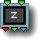23 3D z set

parameters :
A : Z value (-16. : 16.)

discussion :
Use this component to create a third coordinate (whose value is determined by parameter A) that can be passed on to 3D functions or used as a controller value. The incoming x and y values are passed unchanged through the leftmost outputs. Parameter A's value is sent out the rightmost outlet. Animating this parameter is a great way to control solid textures such as 3D noise functions. Use this function at the top of the system if you want to use 33 components near the top of the tree but do not want to use the system's third global input (time) which is incremented with every frame.

##23 3D Axonometric

parameters :
A : Scale X (0. : 2.)
B : Scale Y (0. : 2.)

discussion :
Use this component to create a third coordinate whose value is related to the incoming x,y values. Unlike 3D zset the third output (the z value) is not a constant value but calculated from the x and y values. The scale parameters are multiplied by the x and y inputs before passing them out. The outputs are calculated using the following formulas:

• Left output = Ax
• Middle output = By
• Right output = (Ax + By)/2

##23 Inverse projections #

parameters :
A : Radius (0.50 : 8.)

discussion :
Provides inverse mappings from 2D to 3D spaces. Inverse Sphere 360 is useful to create 3D coordinates for 360 degree environments maps or to create seamless 2D textures that can be projected on a sphere.

algorithms :
• Spherical distort :
Spherical distort is a space transform function that creates a 3D space/object by projecting the incoming space onto a half-sphere. The effect is similar to the image that results from holding a mirrored ball over a picture. The image at left shows the bending of the XY space applied to the logo grid image. The radius parameter controls the size of the half-sphere used for the projection. The smaller the radius is, the greater the resulting space distortion. This is a great component for radiant and supernova-type effects.
• Inverse Sphere 360 :
Maps 2D coordinates of the Sphere to 3D coordinates. Typically the source 2D area will be have a 2:1 ratio and x will map onto the longitudes while y onto the latitudes.
• Inverse Cylindrical 360 :
Maps 2D coordinates of the Cylinder to 3D coordinates. Y is unchanged while X will map into the base circle longitudes.

##23 3D plane

parameters :
A : Banking X (-1.57 : 1.57)
B : Plane Offset (-16. : 16.)
C : Z slope (-3.14 : 3.14)

discussion :
Create a 3D plane on the canvas which can be banked and tilted and returns the 3D XYZ coordinates on the plane. Like all 3D object components, the area outside the object is mapped to infinity (which is painted with the depth cueing color). The components which follow the "plane" component provide the surface's texture. "Banking x" controls the horizontal tilt, "Plane offset" provides an altitude offset, and "z Slope" controls the forward/back tilt.

With all pseudo 3D graphic primitives (2D inputs) the projection into 2D space is done within ArtMatic and theses objects cannot be used as real 3D primitives in Voyager as they are already rendered into 2D canvas when Voyager uses them. They are useful for fast 3D effects within a 2D graphic context. The projection used to render the object in the ArtMatic canvas is a simple implicit perspective projection that can be manipulated with the 22 Scale and offset tile and the 33 translation tile. When 2D coordinates are needed there is a parametric version of the plane (face), the cube and the room. Example: Libraries/Graphic Design/pface and plane.artm

##23 3D sphere

parameters :
A : Offset x (-16. : 16.)
B : Offset y (-16. : 16.)
C : Offset z (-32. : 32.)

discussion :
Renders a pseudo 3D sphere into ArtMatic canvas and returns the 3D XYZ coordinates of the sphere surface. The 'sphere' can be moved along the x, y and/or z axes. Regions outside the sphere are assigned infinity which makes them transparent for most ArtMatic functions. The 'Offset z' parameter controls the sphere offset along the z axis and can be used to scale the object.
See 3D plane above for more details on pseudo 3D objects.

##23 3D ellipsoid

parameters :
A : Radius (0.12 : 24.)
B : Vertical skew (0. : 8.)
C : Offset z (-32. : 32.)

discussion :
Renders a 3D ellipse on the canvas. Outputs are the XYZ coordinates on the surface of the ellipsoid.
See
3D plane for more details on pseudo 3D objects.

##23 3D tube

parameters :
A : Offset x (-16. : 16.)
B : Offset y (-16. : 16.)
C : Radius (0.12 : 24.)

discussion :
Renders a 3D tube whose radius and position can be modified. By default (Offset 0,0) the virtual camera is inside the tube. Cool tunnel effects can be done when animating the z coordinate.
See
3D plane for more details on pseudo 3D objects.

Example: Libraries/Animated Graphics/Techno Tunnel.artm

##23 3D cube

parameters :
A : Angle xy (-6.28 : 6.28)
B : Angle zx (-6.28 : 6.28)
C : Offset z (-32. : 32.)

##23 3D box

parameters :
A : Size y (0.25 : 12.57)
B : Size zx (0.25 : 12.57)
C : Offset z (-32. : 32.)

##23 3D room

parameters :
A : Offset z (-16. : 16.)
B : Angle oY (xz) (-6.28 : 6.28)
C : Room size (0. : 16.)

##23 3D parametric face

parameters :
A : Angle oY (xz) (-π2: π2)
B : Angle oX (yz) (-π2: π2)
C : Offset z (-32. : 32.)
D : Height (-3 : 3)

discussion :
Render a single 3D face and returns its parametric 2D coordinates in (x,y) (parametric coordinates are attached to a face and will moves with the object). The z output will contain the 3D z coordinate. Use this object to perform 3D rotation of your movies and pictures. Regions outside the face are assigned infinity and should be transparent under most Artmatic functions. In RGB mode infinities values are shaded with the depth cue color. The 'Offset z' parameter controls the face offset along the view axis and can be used to scale the object. Unlike the
3D plane which is infinite in size the face has boundaries that are by defaut at +/- π. 'Height' can be set to non zero for other aspect ratios than square.
See 3D plane for more details on pseudo 3D objects.

Example: Libraries/Graphic Design/HumanDots Face.artm

##23 3D parametric cube

parameters :
A : Angle oY (xz) (-π2: π2)
B : Angle oX (yz) (-π2: π2)
C : Offset z (-32. : 32.)
D : Height (-3. : 3.)

##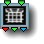23 3D parametric room

parameters :
A : Angle oX (yz) (-π2: π2)
B : Angle oY (xz) (-π2: π2)
C : Room size (0. : 16.)

##23 Perlin noise + derivative

parameters :
A : Amplitude (-8. : 8.)
B : Phase (-32. : 32.)
C : Frequency (-8. : 32.)

##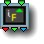23 Looper

parameters :
A : Iterations (0 : 500)
B : Count Multiplier (0. : 4.)

discussion :
Unlike the
Iterations component, the Looper component loops the component(s) connected to its inputs. 'Looper' will evalutate the transform (or transforms) that feed it recursively. After the first time through the loop, the component that directly feeds the looper receives the output from the previous iteration. This process is carried out for each successive iteration until the 'Iterations' count is achieved. When the option "Include Untransformed" is active iteration 0 returns the untransformed space. In mathematical terms if F(v) is the vector transform the 23 looper will compute F(F ...(F(F(v)))) 'Iterations' times.
Output 3 returns the iteration number multiplied by 'Count Multiplier.
In general you will use a 22 Compiled Tree to hold a more complex transform (Compiled trees made up of a few simple space transforms can result in staggeringly complex patterns) but complete Affine transforms like the one provided by 22 Maths tools # can be used directly as well.
As with iterative looped tree a memory component like 11 Memory Logic# or 11 Memory Add is usually needed to accumulate all iterations results. For Fractal transforms you may also use the 21 Orbit Analyzer # tool for various fractal rendering strategies.

##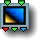23 Shaded plain color #

parameters :
A : Red (0. : 1.)
B : Green (0. : 1.)
C : Blue (0. : 1.)
D : Contrast % (0. : 500.)

algorithms :
• Dual color (legacy):
Negative inputs maps to the complementary color while regions closing to zero are faded to black. The 'Contrast' parameter scales the shading symmetrically around zero.
• Single color contrast:
• Dual color contrast:
Similar to Dual color but the 'Contrast' parameter scales the shading downward in X and symmetrically in Y.
• Dual Aux color A contrast:
Uses Aux Color A for negative x instead of complementary color.
• Dual Aux color B contrast:
Uses Aux Color B for negative x instead of complementary color.
• Dual color ramp:
Uses Aux Color B for negative x instead of complementary color and uses black to shade region near zero x. brightness shading uses uses D*|x|
• Triple color ramp
Uses Aux Color B for negative x instead of complementary color and uses Aux Color A instead of black to shade region near zero x. Practically it gives you a 3 color ramp as a procedural gradient. brightness shading uses uses D*|x|

##23 RGB color shade

parameters :
A : Hue Rotate (0. : 6.28)
B : Saturation (0. : 1.)
C : Luminance Pan x<->y (0. : 1.)

discussion :
Returns a shaded color defined by "Hue Rotate", "Saturation" and "luminance". A mix of x and y inputs modulate the brightness of the result. Use this component to create subtle shadings of a single hue, such as when creating clouds, mist, or earth textures. This component can take input from either a space or two individual inputs.

##23 RGB main gradient

parameters :
A : Scale x (0. : 8.)
B : Scale y (0. : 8.)
C : Gradient Offset (-4. : 4.)

discussion :
Create shaded RGB-color output using the colors of the current ArtMatic gradient. Input x sets the position in the gradient while y shades the resulting color.

Simple 3D shading and lighting effects can be easily created with this shader, especially when the second input is fed by the Derivative or 2D Derivative component.

"Scale x" & "Gradient Offset" determines the gradient mapping from input x to the gradient position. "Scale y" controls the amount of shading applied to the resulting color by scaling the incoming input y values.

Just as with the 21 Ax + By + C, you can use either a 2D space (a component with two outputs) as an input or two independent surfaces.

algorithms :
• Input mix : gradient(x+y) :
The X and Y input values are multiplied by their respective scale parameters and added together in the input mix mode. The resulting sum is mapped to a position on the active gradient. This handy component lets you mix ArtMatic gradient-based colors with RGB-based components.
In the Color mix mode the gradient is applied to X & Y independently and mixed afterwards.

##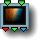23 Shaded main gradient #

parameters :
A : Gradient Scale X (0. : 8.)
B : Shading amount % (0. : 1.)
C : Gradient Offset (-2. : 2.)

discussion :
Create shaded RGB-color output using the colors of the current ArtMatic gradient. The various algorithms gives a greater flexibility on how inputs are affecting the shading of the RGB main gradient. "Gradient Scale X" & "Gradient Offset" determines the gradient mapping from input x to the gradient position."Shading amount " sets how much shading has applied.

If even greater control on the shading is needed you may just use a 13 Main Gradient to generate the color and sets the shading maths yourself prior to multiply it with the color using the 43 w scale or w multiply. Furthermore the 13 version has more option on how the input is mapped to the gradient.

algorithms :
The legacy basic shader where the gradient is multiplied by input y
The gradient is done with the logarithm of (x) and multiplied by input y as well.
This mode corresponds to the Balanced log clut mode for scalar 1D output system. You will need to adjust the gradient scale (parameter A) to get a similar result. It reverts the hues of the gradient for negative x; In addition it shades the result with input y;
• Log mirror, Colored Sines :
This multiplies the gradient (It uses Log mirror for the gradient) with a colored sine wave that uses the Aux color B controlled by input B for average while going from black to while.

When the second input is a packed 3d vector V, it adds another sines controlled by V.y using Aux color C prior the multiply. V.z adds a third sine using the depth cue color. If you don't want that additional light effect, set the depth cue color to black.

• The parameter B sets the amount of shading.
• The parameter D scales the Y input (or packed inputs).

• Procedural D (mul +DC) :
This mode corresponds roughly to the Procedural D clut mode for scalar 1D output system. It uses Log mirror for the gradient. When the second input is a packed 3d vector V, it multiplies with Saw(V.x)*Aux Color A + Saw(V.y)*Aux Color B (Saw is defined by 1-ABS(x)). V.z is used to feed an analog of the global depth cuing. When the second input is scalar it just multiplies the gradient with Saw(y)*Aux Color A

• The parameter B sets the amount of shading.
• The parameter D scales the Y input (or packed inputs)

• Procedural E (add +DC) :
This mode corresponds roughly to the Procedural E clut mode for scalar 1D output system. When the second input is a packed 3d vector V, it adds to the main gradient V.x*Aux Color A + V.y*Aux Color B. V.z is used to feed an analog of the global depth cuing. When the second input is scalar it just adds y*Aux Color A

• The parameter B sets the amount of shading.
• The parameter D scales the Y input (or packed inputs)

• Linear mixed (+DC) :
When the second input is scalar it just multiplies the gradient with y*Aux Color A. When the second input is a packed 3d vector V, it multiplies the main gradient by V.x*Aux Color A and adds. V.y*Aux Color B. V.z is used to feed an analog of the global depth cuing.
• Linear glossy :

##23 Bezier main gradient

parameters :
A : Input range (0.25 : 64.)
B : Offset (-16. : 16.)
C : Output range (0.25 : 64.)

discussion :
This component is similar to the RGB Main Gradient component but it uses bezier curves rather than straight linear interpolation to map input values to the gradient colors. By using bezier curves to interpolate from the gradient to RGB values, the output values are ArtMatic Voyager friendly since each output curve has a continuous derivative.

As a consequence of the bezier interpolation RGB main gradient can be used to implement 3 parallel filters for surface sculpting. Essentially, this component separates the Red, Green and Blue content of the gradient and outputs them as three independent curves that can be used as different terrains filters that would be blended afterwards to create a rich a various topography.

Note: 13 Indexed Gradient and 13 Main Gradient also uses bezier interpolation. The Output range should be left at 1 for color shaders and the Input range can be used to control the input mapping to gradient. Example: individual RGB curves of the bezier gradient.algorithms :
• Input mix : gradient(x+y) :
The X and Y input values are multiplied by their respective scale parameters and added together in the input mix mode. The resulting sum is then mapped to a position on the active gradient.
In the Color mix mode the Bezier Main Gradient is applied to X & Y independently and mixed afterwards.

##23 Indexed gradient

parameters :
A : Gradient Scale X (0. : 2.)
B : Luminance Scale Y (0. : 1.)
C : Gradient Index (0 : 48)

discussion :

Warning: When exporting systems for use in ArtMatic Voyager, this component may return different colors unless you make sure that the other application uses the same gradient library. Use the Gradient Editor to open or export gradient libraries.

##23 Y indexed gradient

parameters :
A : Scale (0. : 2.)
B : Gradient index mod % (0 : 1)

discussion :
This component is a color shader that maps the x-input to a color of the gradient selected by the y-input. This component is similar to RGB Main Gradient except that the y-input selects the gradient to be used.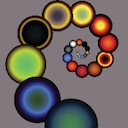The Y-Indexed Gradient component allows a different gradient to be used by each iteration of a looped system when the iteration number is connected to the y-input of Y-Indexed Gradient.

"Scale" is a scaling factor applied to the x-input value. Setting the parameter high makes the component very sensitive to value changes. The y-value picks the gradient from which the color is picked. The current gradient (the one visible above the canvas) is gradient 0. The second gradient in the palette is gradient 1.

"Gradient Index Mod %" determines the mapping of y-input to gradient number. When it is at 1, integer y-values (0, 1, 2, etc.) pick the color from the corresponding gradient in the library.

##23 RGB color gradient

parameters :
A : Angle red (-3.14 : 3.14)
B : Angle green (-3.14 : 3.14)
C : Angle blue (-3.14 : 3.14)

discussion :
Returns a procedural RGB color defined by the sum of a red, a green & a blue ramp, each rotated by the corresponding angle parameters. When the angle are the same it will return grey shades.

The brightness intensifies as XY gets far from zero. The angle are defined in radians but you can type 90 then "d" to convert to degree. A wide variety of vibrant color and lighting effects can be achieved with this simple component.

The color shades obtained by setting the angles to 90 degrees 0 and -90 degrees##23 RGB linear hues

parameters :
A : Hue Rotation (-1. : 1.)
B : Saturation (0. : 1.)
C : Frequency (0. : 4.)

discussion :
Returns a procedural RGB color where X input modulates the hue given by "Hue Rotation" . On the Y input axis when Y is negative then the inverse hue is used. It blends the Y+ and Y- colors around the Y=0 axis.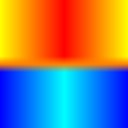##23 RGB radial hues

parameters :
A : Hue Rotation (-1. : 1.)
B : Saturation (0. : 1.)

discussion :
Returns a procedural RGB color by mapping X&Y to a classic color wheel (shown in the component's icon). The two inputs are treated as an x/y coordinate pair. The angular position of the input point (the value pair) determines the hue. Parameter A rotates the color wheel and thus changes the color which corresponds to a particular angle. The Saturation parameter determines the luminance (brightness) of the colors. This component is frequently used to create vibrantly colored surfaces. When this component is used as the color shader, it is as if the original undistorted space (the flat plane) is a sheet of paper with the color wheel printed on it, and the system's space distortions and remapping are really distortions and remapping of the sheet of paper with the color wheel on it. The more chaotic the distortions, the less like a color wheel the image will appear.Example: RGB radial hues is a great color shader to use with fractal space transform as it will color the fractal according to the direction of the escape orbit.##23 RGB concentric hues

parameters :
A : Hue Rotation (-1. : 1.)
B : Saturation (0. : 1.)
C : Frequency (0. : 2.)

discussion :
Returns a procedural RGB color defined as a range of hues that vary with the distance from the origin. The luminosity decreases in inverse proportion to the point's distance from the origin (0,0). Parameter A defines the "base hue" used for shading, and Parameter B controls the color saturation. Parameter C determines the spacing of the hue variations. Luminosity becomes lower as the distance from zero increases (and then starts increasing again).

##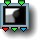23 Max to gradient

parameters :
A : Amplitude X (0. : 2.)
B : Amplitude Y (0. : 2.)

discussion :
This component uses two incoming surfaces to build a color map using their comparaison. Colors are taken from the current
The Amplitudes X and Y parameters scales the lower and upper part of the resulting gradient.
ArtMatic Voyager tip: This component is often used in ArtMatic Voyager systems to create colors for a surface based on the maximum values of the two input functions -- similar to the role served by the "21 Min-Max" component. With it, each "winner" will have its own color.

##23 RGB Pict/Movie

parameters :
A : Size (0. : 4.)
B : Contrast (0. : 2.)
C : Tiling (0. : 1.)

discussion :
This is the true color version of the Pict/Movie component.

##23 RGB Pict/Movie mirror

parameters :
A : Size (0. : 4.)
B : Contrast (0. : 2.)

discussion :
This variant of the color pict/movie component creates mirrored tiles of the picture when the picture is zoomed out.

##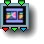23 Pict/Movie Overlay

parameters :
A : Size (0. : 4.)
B : Tile X (0. : 1.)
C : Tile Y (0. : 1.)

discussion :
A variation of the basic pict/movie component that provides independent control of horizontal and vertical tiling. Infinity is generated beyond the picture boundaries. This makes it simple to overlay the output and control the background color (the depth-cueing color).

##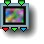23 RGB Multi Perlin random

parameters :
A : Amplitude (-8. : 8.)
B : Octaves (1. : 32.)
C : Frequency (0. : 16.)
D : Roughness (0.25 : 0.75)

discussion :
Sums three 2D Perlin noise functions for each RGB channel with doubled frequencies for each added octave (parameter B). The Perlin noise function which is normally balanced (outputs both negative and positive values) is scaled and offset so that only positive values are generated. When the Amplitude is zero, this component produces a uniform 50% (neutral) gray. Negative amplitude values invert the colors so that color-complements are generated. Amplitude can be seen as a saturation or color contrast control.

algorithms :
• RGB perlin noise (+) :
• Balanced perlin :

##23 RGB Random Fractal

parameters :
A : Color Saturation (0. : 2.)
B : Phase (-16. : 16.)
C : Frequency (0. : 2.)
D : Roughness (0.30 : 0.90)

discussion :
Randomly-generated 2D color texture which is sensitive to the setting of the Max. Iterations for Fractals preference. The amplitude controls the saturation. A great texture for vibrant color textures.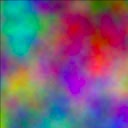##23 RGB MultiFractal

parameters :
A : Amplitude (0. : 2.)
B : Phase (-16. : 16.)
C : Frequency (0. : 16.)

discussion :
This component is a 2D Multi-Fractal RGB noise component. This can be viewed as 3 independent Multi-Fractal functions for each of the RGB channels. The output values are scaled and offset so that only positive values are generated. When the amplitude is zero, the output is a uniform 50% gray. The Amplitude can be seen as a saturation or color contrast control.

##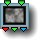23 Lunar rocks

parameters :
A : Amplitude (0. : 2.)
B : Phase (-32. : 32.)
C : Frequency (0. : 16.)
D : Roughness (0.50 : 0.90)

discussion :
Lunar Rocks is a composite 2D color texture providing a color texture reminiscent of random rocky material. The underlying algorithm is similar to the 21 Lunar Rocks component. This primitive is useful for creating natural-looking mineral shading - especially when used in ArtMatic Voyager.

##23 Color Bubbles

parameters :
A : Saturation (0. : 1.)
B : Shade (0. : 1.)
C : Frequency (0. : 8.)

discussion :
Color the 2D space with randomly-sized and colored bubbles/facets using a voronoi tiling algorithm.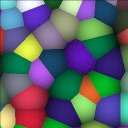##23 String noise

parameters :
A : Thickness (0. : 2.)
B : Hues (0. : 1.)
C : Frequency (0.06 : 8.)

discussion :
Color the 2D space with random colored "strings".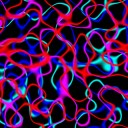##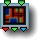23 Techno boxes

parameters :
A : Amplitude (0. : 8.)
B : Skew (0. : 2.)
C : Frequency (0. : 4.)

discussion :
Techno boxes is a decorative RGB shader that generally used to provide surface texture.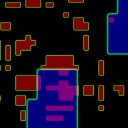##23 Color Pyramids

parameters :
A : Amplitude (0. : 8.)
B : Phase (-16. : 16.)
C : Frequency (0. : 8.)

discussion :
Color the space with randomly-sized and colored pyramids.##23 RGB grass

parameters :
A : Amplitude (0. : 1.)
B : Skew angle (-3.14 : 3.14)
C : Frequency (1. : 32.)

discussion :
RGB Grass is a 2D color texture which evokes - in an impressionistic way - grass seen from afar. This primitive was added primarily for use in ArtMatic Voyager where it is slope dependent (grass tends to appear where the terrain is flat). Within ArtMatic Pro, the "slope values" are derived from the global X input values (since they would be otherwise undefined).

Example: RGB grass as seen in ArtMatic Voyager.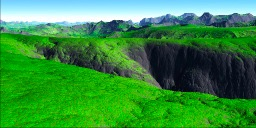##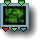23 RGB foliage

parameters :
A : Contrast (0. : 4.)
B : Hues Offset (0. : 1.)
C : Frequency (0. : 32.)

discussion :
RGB foliage is a 2D color texture that impressionistically evokes foliage as seen from afar. The hues offset parameter slides from summer green shades to autumn red shades.

Example: RGB foliage used to texture an ArtMatic Voyager scene.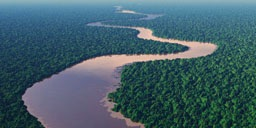##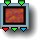23 RGB soft random hues

parameters :
A : Hue Rotation (-1. : 1.)
B : Saturation (0. : 1.)
C : Frequency (0. : 8.)

discussion :
Color the incoming space with random modulations of a narrow range of hues. Naturalistic effects are achieved with a low value for the saturation parameter. At the minimum saturation, only a single hue is left. 'Hue rotation' determines the base color for the variations. "Saturation" determines the average brightness of the resulting colors. 'Frequency' determines the distortion density.

## Packed Mixers Family

The group of components whose names include "packed" is used for mixing packed streams - usually from RGB components or branches. When "infinity" is encountered in one of the inputs, it is treated as transparent by these mixing functions. These functions can accept both packed and unpacked inputs.

##23 Packed XYA logic #

parameters :
A : Smoothness % (0. : 16.)

algorithms :
• Maximum z(alpha):
return the point with maximum z
• Minimum z(alpha):
return the point with minimum z
• Overlay :
Use the "Overlay" logic applied to points. See 21 Logic tools #
• Underlay :
Use the "Underlay" logic applied to points

##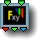23 Packed Vector Maths #

parameters :
A : Amplitude x*y (0. : 2.)
B : Offset y (0. : 2.)
C : Blend % (0. : 1.)

algorithms :
• Blend:
Blend two 3D vectors. Equivalent to RGB crossfade
• Subtract (X-Y):
Substract vector Y from X : (X-Y)
• Difference |X-Y|:
Returns the absolute value for each components of X-Y
• Multiply:
Returns (X*(Y+paramB)) when Blend is at 1; otherwise it blends the multiply with X like the "amount " did in the previous implementation.
• Cross product:
Returns the vector normal to vector X and Y given by the cross product . Interesting color remapping can be done when applied to RGB vectors. The option to clip values below zero is the default. For true vector maths set it to "allow negatives values".
• Dot product :
Returns the dot product of vectors X & Y. Since the dot product returns a scalar value the output color will be a gray level related to the angle of the 2 vectors. The function is also available in packed output form in 21 S:P Maths
• Length :
• Squared length :
Added in Artmatic Engine 8 'Length' and the 'Squared length' version returns the length of vectors X -Y. This will create a circle centered at Y when Y is a constant 3D position. The squared version omits the square root of the length function. When processing a large number of points better use the squared version and do a square root just once at the end as illustrated in this example : Libraries/Maths/Parametrics and motion/Motion parametric 3D balls.artm

##23 Packed RGB Crossfade

parameters :
A : Interpolate (0. : 1.)

##23 Packed RGB Add

parameters :
A : Amplitude A (-2. : 2.)
B : Amplitude B (-2. : 2.)

discussion :
Mix two images (RGB color streams) by adding the values of the merged streams' pixels. The two parameters control the relative contribution of the two images to the final mix. Packed RGB add is equivalent to
21 S:P Maths "Add" algorithm when its passed 2 RGB packed stream. It can also be used to add two 3D vectors (x,y,z).

##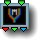23 Packed RGB Max

parameters :
A : Amplitude A (0. : 2.)
B : Amplitude B (0. : 2.)
C : Feather % (0. : 1.)

discussion :
Returns the maximum RGB value for each of the packed inputs. It needs to be fed by packed inputs. The 'Smoothness' parameter will smooth discontinuities if non zero.

##23 Packed RGB Min

parameters :
A : Amplitude A (0. : 2.)
B : Amplitude B (0. : 2.)
C : Feather % (0. : 1.)

discussion :
Returns the minimum RGB value for each of the packed inputs. The 'Smoothness' parameter will smooth discontinuities if non zero.

##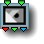23 Packed Depth sort

parameters :
No parameters.

discussion :
This component mixes two 3D objects into a single set of 3D outputs. It is meant to be used with 3D coordinates and not with RGB colors.

Note: This component should always receive its input from Pack components (that receive their input from 3D object components). The algorithm is such that for each set of points received, the set with the lowest value of z wins; in other words, the coordinates closest to the viewer win.

parameters :
A : Scale 0:1
B : Iterations